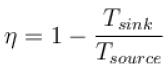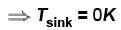Courses

# Second Law Of Thermodynamics NAT Level - 1

## 10 Questions MCQ Test Topic wise Tests for IIT JAM Physics | Second Law Of Thermodynamics NAT Level - 1

Description
This mock test of Second Law Of Thermodynamics NAT Level - 1 for IIT JAM helps you for every IIT JAM entrance exam. This contains 10 Multiple Choice Questions for IIT JAM Second Law Of Thermodynamics NAT Level - 1 (mcq) to study with solutions a complete question bank. The solved questions answers in this Second Law Of Thermodynamics NAT Level - 1 quiz give you a good mix of easy questions and tough questions. IIT JAM students definitely take this Second Law Of Thermodynamics NAT Level - 1 exercise for a better result in the exam. You can find other Second Law Of Thermodynamics NAT Level - 1 extra questions, long questions & short questions for IIT JAM on EduRev as well by searching above.
*Answer can only contain numeric values
QUESTION: 1

### Two ends of a rod are kept at 127ºC and 227ºC. When 2000 cal of heat flows in this rod, then change in entropy (in cal/K) is

Solution: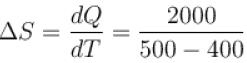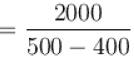ΔS = 20 cal/K

*Answer can only contain numeric values
QUESTION: 2

### One mole of helium and one mole of oxygen contained in two vessels. If temperature of Helium be 300K, what is the temperature of oxygen in Kelvin

Solution:

Entropy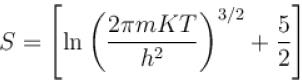of a gas containing N particles is
given as
where m is mass of particle
∴ For the given situation (N = Na)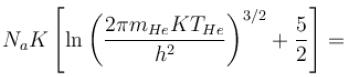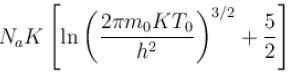∴ m0T0 = mHe THe
⇒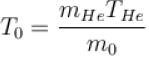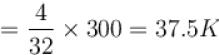*Answer can only contain numeric values
QUESTION: 3

### An engine absorbs heat at temperature of 1000K and rejects heat at 600K. If the engine operates at maximum possible efficiency, the amount of work performed by the amount of work performed by the engine for 200J heat input is (in Joules)

Solution: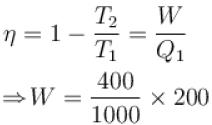=  80J

*Answer can only contain numeric values
QUESTION: 4

1kg of ice melts at 0ºC into water at the same temperature. The change in entropy is (in cal/K)  ____.

Solution:

Change in entropy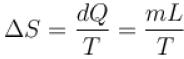L = Latent heat of vapourization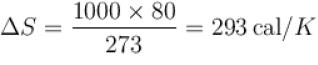*Answer can only contain numeric values
QUESTION: 5

A heat pump working on the Carnot cycle maintains the inside temperature of a house at 22ºC by supplying 450 kJ/s. If the outside temperature is 0ºC, the heat taken, in kJ/s, from the outside air is approximately.

Solution:

The efficiency of Carnot heat engine may be defined as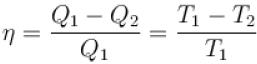Q1 = 450kJ/s, Q2 = ?
T1 = 22 + 273 = 295K
T2 = 273K
Hence,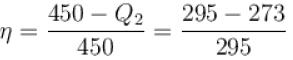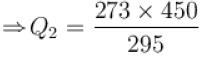= 416.44 kJ/s

*Answer can only contain numeric values
QUESTION: 6

A reversible engine cycle is shown in the following T-S diagram. The efficiency of the engine (in percentage) is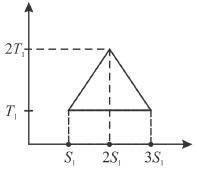Solution:

Available energy per cycle is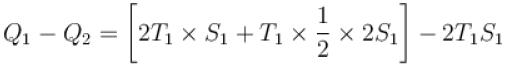= T1 S1
and  Q1 = 3T1 S1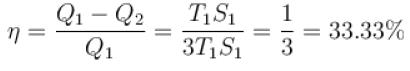*Answer can only contain numeric values
QUESTION: 7

The efficiency of a heat engine working between heat reservoirs at temperature 327ºC and 27ºC respectively (in percentage) is

Solution:

T1 = 327ºC
= 600 K
T2 = 27°C
= 300 K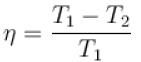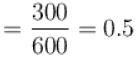⇒ η = 50%

*Answer can only contain numeric values
QUESTION: 8

An amount of heat 1000kJ is transferred from a heat reservoir at temperature TA to another heat reservoir at temperature TB . If TA = 800K and and TB = 500K, what is the change in entropy  ΔS of the combined system in JK-1

Solution:

Conventionally, heat given to the system is taken to be positive and heat extracted from the system is taken to be negative,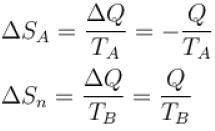ΔS = ΔSA + ΔSB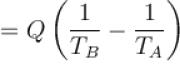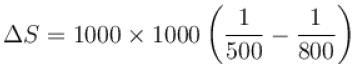ΔS = 750JK-1

*Answer can only contain numeric values
QUESTION: 9

A Carnot engine operating between 27ºC and 127ºC has efficiency equal to (in percentage).

Solution: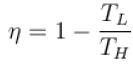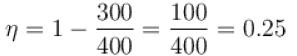⇒ η = 25\%

*Answer can only contain numeric values
QUESTION: 10

For a reversible heat engine, the efficiency can be 100% if the temperature of the sink (in K) is

Solution: